International
Tables for
Crystallography
Volume B
Reciprocal space
Edited by U. Shmueli

International Tables for Crystallography (2006). Vol. B. ch. 1.3, pp. 61-62   | 1 | 2 |

## Section 1.3.4.2.1.7. Sampling theorems, continuous transforms, interpolation

G. Bricognea

aMRC Laboratory of Molecular Biology, Hills Road, Cambridge CB2 2QH, England, and LURE, Bâtiment 209D, Université Paris-Sud, 91405 Orsay, France

#### 1.3.4.2.1.7. Sampling theorems, continuous transforms, interpolation

| top | pdf |

Shannon's sampling and interpolation theorem (Section 1.3.2.7.1) takes two different forms, according to whether the property of finite bandwidth is assumed in real space or in reciprocal space.

 (1) The most usual setting is in reciprocal space (see Sayre, 1952c). Only a finite number of diffraction intensities can be recorded and phased, and for physical reasons the cutoff criterion is the resolution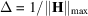. Electron-density maps are thus calculated as partial sums (Section 1.3.4.2.1.3), which may be written in Cartesian coordinates as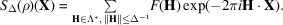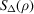is band-limited, the support of its spectrum being contained in the solid sphere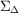defined by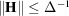. Let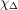be the indicator function of. The transform of the normalized version ofis (see below, Section 1.3.4.4.3.5)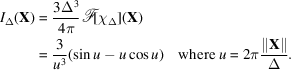By Shannon's theorem, it suffices to calculateon an integral subdivision Γ of the period lattice Λ such that the sampling criterion is satisfied (i.e. that the translates ofby vectors of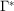do not overlap). Values ofmay then be calculated at an arbitrary point X by the interpolation formula: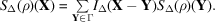(2) The reverse situation occurs whenever the support of the motif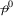does not fill the whole unit cell, i.e. whenever there exists a region M (the molecular envelope'), strictly smaller than the unit cell, such that the translates of M by vectors of r do not overlap and that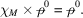It then follows that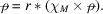Defining the interference function' G as the normalized indicator function of M according to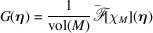we may invoke Shannon's theorem to calculate the value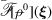at an arbitrary point ξ of reciprocal space from its sample values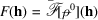at points of the reciprocal lattice as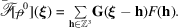This aspect of Shannon's theorem constitutes the mathematical basis of phasing methods based on geometric redundancies created by solvent regions and/or noncrystallographic symmetries (Bricogne, 1974). The connection between Shannon's theorem and the phase problem was first noticed by Sayre (1952b). He pointed out that the Patterson function of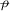, written as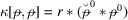, may be viewed as consisting of a motif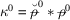(containing all the internal interatomic vectors) which is periodized by convolution with r. As the translates of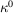by vectors of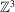do overlap, the sample values of the intensitiesat nodes of the reciprocal lattice do not provide enough data to interpolate intensities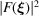at arbitrary points of reciprocal space. Thus the loss of phase is intimately related to the impossibility of intensity interpolation, implying in return that any indication of intensity values attached to non-integral points of the reciprocal lattice is a potential source of phase information.

### ReferencesBricogne, G. (1974). Geometric sources of redundancy in intensity data and their use for phase determination. Acta Cryst. A30, 395–405.Google ScholarSayre, D. (1952b). Some implications of a theorem due to Shannon. Acta Cryst. 5, 843.Google ScholarSayre, D. (1952c). The Fourier transform in X-ray crystal analysis. In Computing methods and the phase problem in X-ray crystal analysis, edited by R. Pepinsky, pp. 361–390. State College: Penn. State University.Google Scholar